Next: Atmospheric Stability Up: Equilibrium of Compressible Fluids Previous: Isothermal Atmosphere

In fact, the temperature of the Earth's atmosphere is not uniform, but instead decreases steadily with increasing altitude. This effect is largely due to the action of convection currents. When a packet of air ascends, under the influence of such currents, the diminished pressure at higher altitudes causes it to expand. Because this expansion generally takes place far more rapidly than heat can diffuse into the packet, the work done against the pressure of the surrounding gas, as the packet expands, leads to a reduction in its internal energy, and, hence, in its temperature. Assuming that the atmosphere is in a continually mixed state, while remaining in approximate vertical force balance (such a state is known as a convective equilibrium), and that the effect of heat conduction is negligible (because the mixing takes place too rapidly for thermal diffusion to affect the temperature), we would expect the adiabatic gas law, (13.4), to offer a much more accurate description of the relationship between atmospheric pressure and density than the isothermal gas law, (13.3).

Let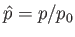,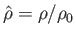, and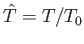, where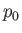,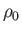, and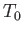are the pressure, mass density, and temperature of the atmosphere, respectively, at ground level. The adiabatic gas law, (13.4), can be combined with the ideal gas equation of state, (13.6), to give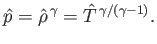(13.11)

The isothermal scale height of the atmosphere is conveniently redefined as [cf., Equation (13.9)](13.12)

Equations (13.5), (13.11), and (13.12) yield(13.13)

where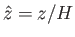, or, from Equation (13.11),(13.14)

The previous equation can be integrated to give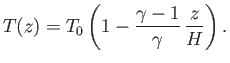(13.15)

It follows that the temperature in an adiabatic atmosphere decreases linearly with increasing altitude at the rate of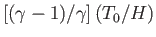degrees per meter. This rate is known as the adiabatic lapse rate of the atmosphere. Using the values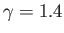,, and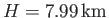, which are typical of the Earth's atmosphere, we estimate the lapse rate to be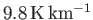. In reality, the lapse rate only takes this value in dry air. In moist air, the lapse rate is considerably reduced because of the latent heat released when water vapor condenses.

Equations (13.11) and (13.15) yield(13.16)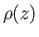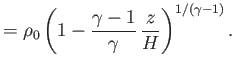(13.17)

Because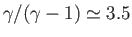and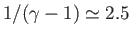, it follows that pressure decreases more rapidly than density in an adiabatic atmosphere. Moreover, the previous three equations imply that an adiabatic atmosphere has a sharp upper boundary at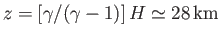. At this altitude, the temperature, pressure, and density all fall to zero. Of course, above this altitude, the temperature, pressure, and density remain zero (because they cannot take negative or imaginary values). In contrast, an isothermal atmosphere has a diffuse upper boundary in which the pressure and density never fall to zero, even at extreme altitudes. It should be noted that, in reality, the Earth's atmosphere does not have a sharp upper boundary, because the adiabatic gas law does not hold at very high altitudes.Next: Atmospheric Stability Up: Equilibrium of Compressible Fluids Previous: Isothermal Atmosphere
Richard Fitzpatrick 2016-03-31